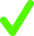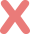Angle A = º Angle B = º Angle C = º Angle A + Angle B + Angle C = º Angle 1 = º Angle 2 = º Angle 3 = º Angle C + Angle 2 = º
00ix

Drag the vertices to change the shape of the triangle.